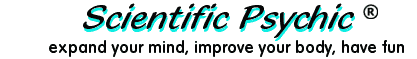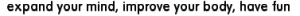Index# The Jewelry BoxAt an eBay auction, a woman's ring and a filigree jewelry box with a hand-painted ceramic top are on sale for \$200.00 Dollars. The jewelry box is valued at \$190.00 Dollars more than the ring. How much is the ring worth?

If you said \$10.00 Dollars, you are wrong! It is not that simple.

This is a problem that requires setting up two equations with two unknowns to find the answer.

The box (B) plus the ring (R) cost \$200.00 Dollars:
B + R = 200
The jewelry box is valued at \$190.00 Dollars more than the ring, i.e., the price of the box (B) is equal to the price of the ring (R) plus \$190.00 Dollars:
B = R + 190
Now we can substitute the right side of the second equation in the first equation and solve for R.
R + 190 + R = 200
2 R = 200 - 190
2 R = 10
R = 10/2
R = 5
The ring is worth \$5.00 Dollars.

The price of the box is \$190.00 plus \$5.00, which is \$195.00 Dollars, and the box plus the ring add up to \$200.00. So, everything checks out.